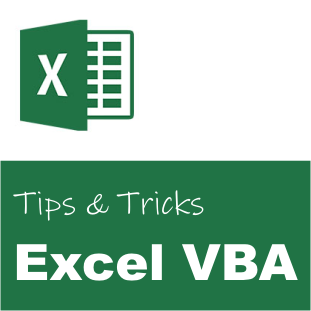# Excel VBA: Force full Calculation of Formulas# Excel VBA: Force full Calculation of Formulas

Have you ever experienced that Excel does not calculate all formulas correctly? I guess we have all experienced extremely large Excel spreadsheets with a lot of formulas. Now and then Excel refuses to calculate all the cell values. I don’t really know why, but it happens more frequently than I would hope for. One way to solve this issue, is to force Excel to re-calculate everything using VBA.

The magic to ensure that everything is calculated is to use three VBA methods:

1. Application.Calculate

Calculates all open workbooks.

2. Application.CalculateFull

Forces a full calculation of the data in all open workbooks.

3. Application.CalculateFullRebuild

For all open workbooks, forces a full calculation of the data and rebuilds the dependencies. Dependencies are the formulas that depend on other cells.

You will find the links to the Microsoft user guides for the above methods in the “Related” section below.

### Code

```Option Explicit

' Force Full Calculation
Public Sub ForceFullCalculation()
On Error Resume Next

' Normal calculation
Call Application.Calculate

' Extended calculation
Call Application.CalculateFull

' Full Calculation
Call Application.CalculateFullRebuild
End Sub```

### Usage

The code can be executed in the following way:

```' Perform full calculation of the data and rebuilds the dependencies.
Call ForceFullCalculation()```

I usually add a Button or a Shape to the main sheet to allow the user to easily force a full calculation of the Workbook. Just keep in mind that the method above re-calculates all open Workbooks. You could consider replacing “Application” with “Application.ThisWorkbook” to recalculate only the current Workbook, i.e. where you have added the custom button.

#### Custom Button

To add a custom button you need to

1. Enable the Developer ribbon.
2. Open the Visual Basic editor (Developer > Visual Basic).
3. Double-click on Sheet1 and paste the code above, i.e. the full code of “Public Sub ForceFullCalculation()”.
4. Press Developer > Controls > Insert > Form Controls > Button
5. In the “Assign Macro” dialog, create a new click event handler, or select the method “Sheet1.ForceFullCalculation”.

In this case you could also have used a ActiveX Command Button. Then you would not have assigned a macro, but instead you would have added “Call ForceFullCalculation()” in the Click event.

#### Custom Shape

If you want a fancier button, you can use a custom shape instead. Follow step 1 to 3 above. Instead of adding a button, press Insert > Shapes and select the shape you want. When added, right-click the shape, and select “Assign Macro…“. Then follow step 5 above to assign the ForceFullCalculation macro to the Click action.